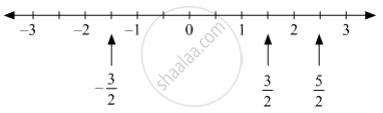# Show the Following Numbers on a Number Line. Draw a Separate Number Line for Each Example. 3 2 , 5 2 , − 3 2 - Mathematics

Sum

Show the following numbers on a number line. Draw a separate number line for each example.

3/2 , 5/2 , -3/2

#### Solution

3/2 , 5/2 , -3/2 can be represented on the number line as follows.Concept: Rational Numbers on a Number Line
Is there an error in this question or solution?

#### APPEARS IN

Balbharati Mathematics 8th Standard Maharashtra State Board
Chapter 1 Rational and Irrational numbers
Practice Set 1.1 | Q 1.1 | Page 2